### Example 35.1 Principal Component Analysis

This example analyzes socioeconomic data provided by Harman (1976). The five variables represent total population (Population), median school years (School), total employment (Employment), miscellaneous professional services (Services), and median house value (HouseValue). Each observation represents one of twelve census tracts in the Los Angeles Standard Metropolitan Statistical Area.

You conduct a principal component analysis by using the following statements:

data SocioEconomics;
input Population School Employment Services HouseValue;
datalines;
5700     12.8      2500      270       25000
1000     10.9      600       10        10000
3400     8.8       1000      10        9000
3800     13.6      1700      140       25000
4000     12.8      1600      140       25000
8200     8.3       2600      60        12000
1200     11.4      400       10        16000
9100     11.5      3300      60        14000
9900     12.5      3400      180       18000
9600     13.7      3600      390       25000
9600     9.6       3300      80        12000
9400     11.4      4000      100       13000
;

proc factor data=SocioEconomics simple corr;
run;


You begin with the specification of the raw data set with 12 observations. Then you use the DATA= option in the PROC FACTOR statement to specify the data set in the analysis. You also set the SIMPLE and CORR options for additional output results, which are shown in Output 35.1.2 and Output 35.1.3, respectively.

By default, PROC FACTOR assumes that all initial communalities are 1, which is the case for the current principal component analysis. If you intend to find common factors instead, use the PRIORS= option or the PRIORS statement to set initial communalities to values less than 1, which results in extracting the principal factors rather than the principal components. See Example 35.2 for the specification of a principal factor analysis.

For the current principal component analysis, the first output table is displayed in the Output 35.1.1.

Output 35.1.1: Principal Component Analysis: Number of Observations

 Five Socioeconomic Variables See Page 14 of Harman: Modern Factor Analysis, 3rd Ed Principal Component Analysis

The FACTOR Procedure

Input Data Type Raw Data 12 12 12

In Output 35.1.1, the input data type is shown to be raw data. PROC FACTOR also accepts other data type such as correlations and covariances. See Example 35.4 for the use of correlations as input data. For the current raw data set, PROC FACTOR reads in 12 records and all these 12 records are used. When there are missing values in the data set, these two numbers might not match due to the dropping of the records with missing values. The last row of the table shows that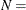12 is used in the significance tests conducted in the analysis.

The SIMPLE option specified in the PROC FACTOR statement generates the means and standard deviations of all observed variables in the analysis, as shown in Output 35.1.2.

Output 35.1.2: Principal Component Analysis: Simple Statistics

Means and Standard Deviations from
12 Observations
Variable Mean Std Dev
Population 6241.667 3439.9943
School 11.442 1.7865
Employment 2333.333 1241.2115
Services 120.833 114.9275
HouseValue 17000.000 6367.5313

The ranges of means and standard deviations for the analysis are quite large. Variables are measured on quite different scales. However, this is not an issue because PROC FACTOR basically analyzes the standardized scales (that is, the correlations) of the variables.

The CORR option specified in the PROC FACTOR statement generates the output of the observed correlations in Output 35.1.3.

Output 35.1.3: Principal Component Analysis: Correlations

Correlations
Population School Employment Services HouseValue
Population 1.00000 0.00975 0.97245 0.43887 0.02241
School 0.00975 1.00000 0.15428 0.69141 0.86307
Employment 0.97245 0.15428 1.00000 0.51472 0.12193
Services 0.43887 0.69141 0.51472 1.00000 0.77765
HouseValue 0.02241 0.86307 0.12193 0.77765 1.00000

The correlation matrix shown in Output 35.1.3 is analyzed by PROC FACTOR.

The first step of principal component analysis is to look at the eigenvalues of the correlation matrix. The larger eigenvalues are extracted first. Because there are five observed variables, five eigenvalues can be extracted, as shown in Output 35.1.4.

Output 35.1.4: Principal Component Analysis: Eigenvalues

Eigenvalues of the Correlation Matrix: Total
= 5 Average = 1
Eigenvalue Difference Proportion Cumulative
1 2.87331359 1.07665350 0.5747 0.5747
2 1.79666009 1.58182321 0.3593 0.9340
3 0.21483689 0.11490283 0.0430 0.9770
4 0.09993405 0.08467868 0.0200 0.9969
5 0.01525537   0.0031 1.0000

In Output 35.1.4, the two largest eigenvalues are 2.8733 and 1.7967, which together account for 93.4% of the standardized variance. Thus, the first two principal components provide an adequate summary of the data for most purposes. Three components, which explain 97.7% of the variation, should be sufficient for almost any application. PROC FACTOR retains the first two components on the basis of the eigenvalues-greater-than-one rule since the third eigenvalue is only 0.2148.

To express the observed variables as functions of the components (or factors, in general), you consult the factor loading matrix as shown in Output 35.1.5.

Output 35.1.5: Principal Component Analysis: Factor Pattern

Factor Pattern
Factor1 Factor2
Population 0.58096 0.80642
School 0.76704 -0.54476
Employment 0.67243 0.72605
Services 0.93239 -0.10431
HouseValue 0.79116 -0.55818

The factor pattern is often referred to as the factor loading matrix in factor analysis. The elements in the loading matrix are called factor loadings. There are at least two ways you can interpret these factor loadings. First, you can use this table to express the observed variables as functions of the extracted factors (or components, as in the current analysis). Each row of the factor loadings tells you the linear combination of the factor or component scores that would yield the expected value of the associated variable. Second, you can interpret each loading as a correlation between an observed variable and a factor or component, provided that the factor solution is an orthogonal one (that is, factors are uncorrelated), such as the current initial factor solution. Hence, the factor loadings indicate how strongly the variables and the factors or components are related.

In Output 35.1.5, the first component (labeled Factor1) has large positive loadings for all five variables. Its correlation with Services (0.9324) is especially high. The second component is basically a contrast of Population (0.8064) and Employment (0.7261) against School (–0.5448) and HouseValue (–0.5582), with a very small loading on Services (–0.1043).

The total variance explained by the two components are shown in Output 35.1.6.

Output 35.1.6: Principal Component Analysis: Total Variance Explained by Factors

Variance Explained by Each
Factor
Factor1 Factor2
2.8733136 1.7966601

The first and second component account for 2.8733 and 1.7967, respectively, of the total variance of 5. In the initial factor solution, the total variance explained by the factors or components are the same as the eigenvalues extracted. (Compare the total variance with the eigenvalues shown in Output 35.1.4.) Due to the dropping of the less important components, the sum of these two numbers is 4.6700, which is only a little bit less than total variance 5 of the original correlation matrix.

You can also look at the variance explained by the two components for each observed variables in Output 35.1.7.

Output 35.1.7: Principal Component Analysis: Final Communality Estimates

Final Communality Estimates: Total = 4.669974
Population School Employment Services HouseValue
0.98782629 0.88510555 0.97930583 0.88023562 0.93750041

In Output 35.1.7, the final communality estimates show that all the variables are well accounted for by the two components, with final communality estimates ranging from 0.8802 for Services to 0.9878 for Population. The sum of the communalities is 4.6700, which is the same as the sum of the variance explained by the two components, as shown in Output 35.1.6.

#### Principal Component Analysis by PROC FACTOR and PROC PRINCOMP

The principal component analysis by PROC FACTOR emphasizes how the principal components explain the observed variables. The factor loadings in the factor pattern as shown in Output 35.1.5 are the coefficients for combining the factor/component scores to yield the observed variable scores when the expected error residuals are zero. For example, the predicted standardized value of Population given the factor/component scores for Factor1 and Factor2 is given by: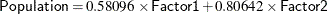If you are primarily interested in getting the component scores as linear combinations of the observed variables, the factor loading matrix table is not the right one for you. However, you might request the standardized scoring coefficients by adding the SCORE option in the FACTOR statement:

proc factor data=SocioEconomics n=5 score;
run;


In the preceding PROC FACTOR statement, N=5 is specified for retaining all five components. This is done for comparing the PROC FACTOR results with those of PROC PRINCOMP, which is described later. The SCORE option requests the display of the standardized scoring coefficients, which are shown in Output 35.1.8.

Output 35.1.8: Principal Component Analysis: Scoring Coefficients for Computing Component Scores

Standardized Scoring Coefficients
Factor1 Factor2 Factor3 Factor4 Factor5
Population 0.20219065 0.44884459 0.1284067 0.64542101 5.58240225
School 0.26695219 -0.3032049 1.48611655 -1.1184573 1.41573501
Employment 0.23402646 0.40410834 0.53496241 0.07255759 -5.6513542
Services 0.32450082 -0.0580552 -1.432726 -1.5828806 -0.0010006
HouseValue 0.27534803 -0.3106762 -0.3012889 2.41418899 -0.6673445

In Output 35.1.8, each factor/component is expressed as a linear combination of the standardized observed variables. For example, the first principal component or Factor1 is computed as: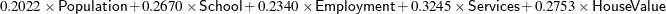Again, when applying this formula you must use the standardized observed variables (with means 0 and standard deviations 1), but not the raw data.

Apart from some scaling differences, the set of scoring coefficients obtained from PROC FACTOR are equivalent to those obtained from PROC PRINCOMP, as specified by the following statement:

proc princomp data=SocioEconomics;
run;


PROC PRINCOMP displays the scoring coefficients as eigenvectors, which are shown in Output 35.1.9.

Output 35.1.9: Principal Component Analysis by PROC PRINCOMP: Eigenvectors

Eigenvectors
Prin1 Prin2 Prin3 Prin4 Prin5
Population 0.342730 0.601629 0.059517 0.204033 0.689497
School 0.452507 -.406414 0.688822 -.353571 0.174861
Employment 0.396695 0.541665 0.247958 0.022937 -.698014
Services 0.550057 -.077817 -.664076 -.500386 -.000124
HouseValue 0.466738 -.416429 -.139649 0.763182 -.082425

For example, to get the first principal component score, you use the following formula: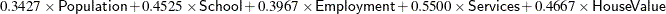This formula is not exactly the same as the one shown by using PROC FACTOR. All scoring coefficients in PROC FACTOR are smaller, approximately a factor of 0.59 to those coefficients obtained from PROC PRINCOMP. The reason for the scalar difference is that PROC FACTOR assumes all factors/components to have variance of 1, while PROC PRINCOMP creates components that have variances equal to the eigenvalues. You can do a simple rescaling of the standardized scoring coefficients obtained from PROC FACTOR so that they match the associated eigenvectors from the PROC PRINCOMP. Basically, you need to rescale each column of the standardized scoring coefficients obtained from PROC FACTOR to have the sum of squares equaling one, which is a defining characteristic of eigenvectors. This could be accomplished by dividing each coefficient by the square root of the corresponding column sum of squares.

For the present example, you can use PROC STDIZE to do the rescaling, as shown in the following statements:

proc factor data=SocioEconomics n=5 score;
ods output StdScoreCoef=Coef;
run;

proc stdize method=ustd mult=.44721 data=Coef out=eigenvectors;
Var Factor1-Factor5;
run;

proc print data=eigenvectors;
run;


First, you create an output set Coef for the standardized scoring coefficients by the ODS OUTPUT statement. Note that StdScoreCoef is the ODS table that contains the standardized scoring coefficients as shown in Output 35.1.8. (See Table 35.3 for all ODS table names for PROC FACTOR.) Next, you use METHOD=USTD in the PROC STDIZE statement to divide the output coefficients by the corresponding uncorrected (for mean) standard deviations. The following formula shows the relationship between the uncorrected standard deviation and the sum of squares: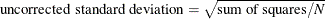Recall that what you intend to divide from each coefficient is its square root of the corresponding column sum of squares. Therefore, to adjust for what PROC STDIZE does using METHOD=USTD, you have to multiply each variable by a constant term of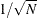in the standardization. For the current example, this constant term is 0.44721 (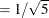) and is specified through the MULT= option in the PROC STDIZE statement. With the OUT= option, the rescaled scoring coefficients are saved in the SAS data set eigenvectors. The printout of the data set in Output 35.1.10 shows the rescaled standardized scoring coefficients obtained from PROC FACTOR.

Output 35.1.10: Rescaled Standardized Scoring Coefficients

Obs Variable Factor1 Factor2 Factor3 Factor4 Factor5
1 Population 0.34272761 0.60162443 0.05951667 0.20403109 0.68949172
2 School 0.45250304 -0.4064112 0.68881691 -0.3535678 0.17485977
3 Employment 0.39669158 0.54166065 0.24795576 0.02293697 -0.6980081
4 Services 0.5500521 -0.0778162 -0.6640703 -0.5003817 -0.0001236
5 HouseValue 0.4667346 -0.4164256 -0.1396478 0.76317568 -0.0824248

As you can see, these standardized scoring coefficients are essentially the same as those obtained from PROC PRINCOMP, as shown in Output 35.1.9. This example shows that principal component analyses by PROC FACTOR and PROC PRINCOMP are indeed equivalent. PROC PRINCOMP emphasizes more the linear combinations of the variables to form the components, while PROC FACTOR expresses variables as linear combinations of the components in the output. If a principal component analysis of the data is all you need in a particular application, there is no reason to use PROC FACTOR instead of PROC PRINCOMP. Therefore, the following examples focus on common factor analysis for which that you can apply only PROC FACTOR, but not PROC PRINCOMP.